# Tutorial to the R-package Luminsescence

## 1. R and RStudio

R is a free software environment for statistical computing and graphics. R provides a wide variety of statistical and graphical techniques, and is highly extensible. R is available as Free Software under the terms of the Free Software Foundation's GNU General Public License in source code form. Many users think of R as a statistics system. We prefer to think of it of an environment within which statistical techniques are implemented. R can be extended (easily) via packages (http://www.r-project.org/).
CRAN, the “Comprehensive R Archive Network” is a collection of sites which carry identical material, consisting of the R distribution(s), the contributed extensions, documentation for R, and binaries. The CRAN master site is at TU Wien, Austria. From CRAN, you can obtain the latest official release of R and a wealth of additional contributed code. CRAN also provides access to documentation on R, existing mailing lists and the R Bug Tracking system (http://www.r-project.org/).
RStudio RStudio is a free and open source integrated development environment (IDE) for R. Like R, RStudio is available under a free software license (http://www.rstudio.org/docs/about). RStudio allows to comfortably use R, manage own code, the workspace, files and folders, plots, packages and help. RStudio further allows managing different projects and code designs.

## 2. Using R as a simple calculator

### Syntax, important symbols

For the first few minutes let us just use the R console (the bottom-left window in RStudio). This is the direct input window, providing access to R. Normally, the console should wait for your commands by showing the prompt (>). Let us start with some important information about the syntax of R and important symbols.

Help may be one of the first and most important issues you desire during the first runs in R. If you know the name of a function but nothing about usage, parameters and output, just type a questionmark and the function name to open the documentation page.

``````?quantile
``````

Did you know that there are at least 9 approaches to calculate quantiles?

Comments, the only thing that may keep your code understandable - even to yourself. Comments are introduced by a hash, one hash for each line of comment. Comments should be used extensively when writing own functions (cf. XXX). Someone (maybe you in a few years) must be able to understand the structure, reason and executed tasks of a function.

``````# A one-line comment

# The beginning of a two-line comment,
# and the second line of this comment.
``````

Operators are usually used to connect numbers or variables, i.e. to process mathematical terms. There are of course more than additions, subtractions, multiplications, divisions, power operations etc. It is common to separate operators by spaces. In the simplest case, R may be used as simple calculator for primitive tasks using numbers and operators:

``````1 + 2  # addition
``````
``````##  3
``````
``````1 - 2  # subtraction
``````
``````##  -1
``````
``````1 * 2  # multiplication
``````
``````##  2
``````
``````1/2  # division
``````
``````##  0.5
``````
``````1^2  # exponentiation
``````
``````##  1
``````
``````sqrt(2)  # root extraction
``````
``````##  1.414
``````

The last example already takes us to what R is all about: using functions to manipulate data.

Functions are the central part in R. A function is an expression that evaluates and returns one or more arguments. A function is usually of the following form:

FUNCTIONNAME(ARGUMENT1 = VALUE1, ARGUMENT2 = VALUE2)

Looks like using functions in R is similar to using functions in other spreadsheet software (MS Excel, Libre Calc). To call a function, its name has to be typed, followed by brackets. Inside the brackets the function arguments may be specified.
To evaluate the square root of 2, one may use

``````sqrt(2)
``````
``````##  1.414
``````

Apart from directly evaluating operations or functions it would be useful to temporarily store the results in variables.

Assigning values to variables should be done using the assign-operators: “<-” or “->”. Although it is also possible to exchange “<-” by “=”, the latter symbol should be reserved to assign function arguments, not function results! There is a shortcut to write “<-”: Alt + -. Assigning values shoul not be a too big deal, as the following examples illustrate.

``````x <- 2  # assign the value 2 to the variable x
y <- sqrt(x)  # evaluate the square root of x and assign it to the variable y
y  # call the variable y
``````
``````##  1.414
``````

Variables are temporary data storage objects or, also, objects with variable value content. They may contain one or a series of unique values. Hence, calling one variable could yield a few thousand values. Using variables may therefore be an efficient way to manage large amounts of data.

Sequences, repetitions, and concatenations may be generated by the functions `seq()` `rep()` and `c()`. Sequences are important constructs for many purposes, which will be introduced later on. So this section may stand here a little bit out of phase but it is worth remembering it.

``````# call documentation for the function seq(): ?seq
x <- seq(from = 1, to = 10)  # create a sequence from 1 to 10
x
``````
``````##    1  2  3  4  5  6  7  8  9 10
``````
``````x <- seq(1, 10)  # the same but shorter
x
``````
``````##    1  2  3  4  5  6  7  8  9 10
``````
``````x <- 1:10  # the same but even shorter
x
``````
``````##    1  2  3  4  5  6  7  8  9 10
``````
``````x <- seq(2, 10, by = 2)  # create a sequence of multiples of 2
x
``````
``````##   2  4  6  8 10
``````
``````x <- seq(10, 2, by = -2)  # the same but in the other direction
x
``````
``````##  10  8  6  4  2
``````
``````x <- seq(2, 10, length.out = 5)
x
``````
``````##   2  4  6  8 10
``````

Of similar importance is the function `rep()`. It repeats sequences of values.

``````# call documentation for the function rep(): ?rep
x <- 1:10  # create a sequence from 1 to 10
y1 <- rep(x, times = 2)
y1
``````
``````##    1  2  3  4  5  6  7  8  9 10  1  2  3  4  5  6  7  8  9 10
``````
``````y2 <- rep(x, each = 2)
y2
``````
``````##    1  1  2  2  3  3  4  4  5  5  6  6  7  7  8  8  9  9 10 10
``````
``````y3 <- rep(x, length.out = 20)
y3
``````
``````##    1  2  3  4  5  6  7  8  9 10  1  2  3  4  5  6  7  8  9 10
``````

It is of course possible to combine and enclose functions and expressions.

``````x <- rep(seq(2, 10, by = 2), 2)  # repeat a sequence two times
x
``````
``````##    2  4  6  8 10  2  4  6  8 10
``````

Concatenating values or variable contents is also a very common task. This is done by the function `c()`.

``````x1 <- seq(1, 10)  # create a sequence
x2 <- seq(11, 20)  # create a second sequence
y <- c(x1, x2)  # concatenate both sequences
y
``````
``````##    1  2  3  4  5  6  7  8  9 10 11 12 13 14 15 16 17 18 19 20
``````
``````y <- c(rep(1, 10), seq(1, 50, by = 5))  # concatenate two other sequences
y
``````
``````##    1  1  1  1  1  1  1  1  1  1  1  6 11 16 21 26 31 36 41 46
``````

## 3. Using R as a bit more sophisticated calculator

### Data types

As in other calculus programs there are different data types. R supports the following atomar (no further subdivision possible) data types:

• `NULL`, empty set.
• `logical`, contains either TRUE (1) or FALSE (0).
• `numeric`, both integer and real numbers. Numeric values are stored integer or double, depending on their accuracy.
• `complex`, complex numbers, consisting of a real and an imaginary part.
• `character`, letters and other strings. They are specified in quotes, e.g. `"Hello"` or `'Hello'`.

It is important to know (about) data types because each data type allows special operations and contains specific information. It is possible to add numeric, complex and logical values but not character values.

``````x <- 1 < 0  # evaluate the inequation
x
``````
``````##  FALSE
``````
``````x <- !x  # negate the current state of x, i.e. convert FALSE to TRUE
x
``````
``````##  TRUE
``````
``````y <- 3 * x  # multiply the values of x (TRUE)
y
``````
``````##  3
``````
``````x <- sum(1, 2)  # calculate the sum of two concatenated numeric values
x
``````
``````##  3
``````
``````x <- sum("1", "2")  # try to calculate the sum of two concatenated character values
``````
``````## Error: ungültiger 'type' (character) des Argumentes
``````
``````x <- sum(as.numeric(x))  # calculate the sum of values, converted to numerics
x
``````
``````##  3
``````

The last example shows how it is possible to convert data types into each other, if this is possible or reasonable. Other functions may be used in analogy: `as.logical()`, `as.numeric()`, `as.complex()`, `as.character()`.

Checking a data type may be also useful. This can be done using `is.logical()`, `is.numeric()`, `is.complex()`, `is.character()`. These expressions return a logical value.

``````x <- 1
is.character(x)
``````
``````##  FALSE
``````
``````x <- as.character(x)
x
``````
``````##  "1"
``````
``````is.character(x)
``````
``````##  TRUE
``````

### Data structures

Since variables can hold more than one unique value, there is of course the possibility (and necessity) to organise or arrange data in variables. This is what data structures is referred to in R. The most common data structures in R are vectors, matrices, arrays, lists, data frames and S4-objects. The latter one is outstanding but included here since it is used in the package Luminescence.
To infer the structure of an arbitrary variable the function `str()` is essential and should always be kept in mind.

#### Vectors

Vectors are perhaps familiar data structures. Vectors contain m rows of values, organised in one column (m:1 structure). Vectors may be of any kind of data type. However, the data type must be the same for all values of the vector. Scalars (one-dimensional data types) do not exist in R, they are represented by a vector with only one row (1:1 structure). The simplest way to create (or expand) a vector is using the function concatenate (`c()`). To infer the number of values in a vector, its length, the function `length()` can be used.

``````x <- 1  # create a numeric vector with one element (a scalar)
str(x)
``````
``````##  num 1
``````
``````length(x)
``````
``````##  1
``````
``````x <- c(x, seq(2, 10))  # enlarge the vector by concatenating a sequence from 2 to 10
str(x)
``````
``````##  num [1:10] 1 2 3 4 5 6 7 8 9 10
``````
``````length(x)
``````
``````##  10
``````
``````x <- as.character(x)
str(x)
``````
``````##  chr [1:10] "1" "2" "3" "4" "5" "6" "7" "8" "9" ...
``````

Arithmetics with vectors follow the usual mathematical rules, i.e. primitive operations with a constant are done component-wise.

``````x <- c(1, 2, 3)  # create a vector
x + 10  # add 10 to (all components of) the vector
``````
``````##  11 12 13
``````
``````x * 2  # multiply (all components of) the vector by 2
``````
``````##  2 4 6
``````

Arithmetics with two vectors also follow usual mathematical rules.

``````x <- c(1, 2, 3)  # create vector x
y <- c(2, 3, 4)  # create vector y
x + y  # calculate vector sum
``````
``````##  3 5 7
``````
``````x * y  # multiply vectors component-wise
``````
``````##   2  6 12
``````
``````x %*% y  # evaluate the inner product, i.e. scalar product
``````
``````##      [,1]
## [1,]   20
``````
``````t(x)  # transpose vector x
``````
``````##      [,1] [,2] [,3]
## [1,]    1    2    3
``````

#### Matrices

Adding one dimension to vectors results in matrices. Matrices contain m rows and n columns of data (m:n structure). The structure of a matrix is perhaps best illustrated by reminding an ordinary spreadsheet (MS Ecxel, LibreCalc) with rows and columns. Matrices can be of any data type but again, the data type must be consistent throughout the data set. Matrices can be created by the function `matrix()` or by concatenating vectors (rowwise or columnwise using `rbind()` and `cbind()`). To infer the number of values (product of rows and columns) the function `length()` can be used. To get the number of rows or columns use `nrow()` and `ncol()`.

``````x <- rep(10, 3)  # create a 3:1 vector
X <- matrix(seq(1, 9), nrow = 3, ncol = 3)  # create a simple 3:3 matrix
X
``````
``````##      [,1] [,2] [,3]
## [1,]    1    4    7
## [2,]    2    5    8
## [3,]    3    6    9
``````
``````str(X)
``````
``````##  int [1:3, 1:3] 1 2 3 4 5 6 7 8 9
``````
``````nrow(X)  # get number of rows
``````
``````##  3
``````
``````ncol(X)  # get number of columns
``````
``````##  3
``````
``````length(X)  # get length of the matrix
``````
``````##  9
``````
``````cbind(X, x)  # concatenate X and x column-wise
``````
``````##             x
## [1,] 1 4 7 10
## [2,] 2 5 8 10
## [3,] 3 6 9 10
``````
``````rbind(X, x)  # concatenate X and x row-wise
``````
``````##   [,1] [,2] [,3]
##      1    4    7
##      2    5    8
##      3    6    9
## x   10   10   10
``````

It is possible and useful to specify row- and column-names for a matrix. This is done by the functions `rownames()` and `colnames`.

``````rownames(X) <- c(1, 2, 3)  # specify matrix row-names
colnames(X) <- c("A", "B", "C")  # specify matrix column-names
X
``````
``````##   A B C
## 1 1 4 7
## 2 2 5 8
## 3 3 6 9
``````

#### Arrays

Adding another dimension to vectors results in arrays. Arrays contain m rows, n columns and o pages of data(m:n:o structure). The structure of an array is perhaps best illustrated by thinking of a Rubik's cube (http://en.wikipedia.org/wiki/Rubik%27s_Cube). Arrays can be of any data type, used in a consistent way. Arrays can be created by the function `array()`. In contrast to matrices, the dimension of the array must be specified explicitly.

``````A <- array(1:8, dim = c(2, 2, 2))
str(A)
``````
``````##  int [1:2, 1:2, 1:2] 1 2 3 4 5 6 7 8
``````
``````A
``````
``````## , , 1
##
##      [,1] [,2]
## [1,]    1    3
## [2,]    2    4
##
## , , 2
##
##      [,1] [,2]
## [1,]    5    7
## [2,]    6    8
``````

It is possible and useful to specify row-, column- and page-names for an array. This is done by the function `dimnames()` which must contain a list with names for each dimension.

#### Lists

A very flexible data structure are lists. They can contain different data structures, which of course have to be consistent for themselves. All contained data structures can be of different length and dimensions. Lists may contain vectors, matrices, other lists and so on. Lists can be created with the function `list()`.

``````info <- "A list with three colours and their rgb-codes."
colours <- c("red", "yellow", "green")  # create a character vector
colcode <- matrix(c(1, 1, 0, 0, 1, 1, 0, 0, 0), ncol = 3)  # create a numeric matrix
collist <- list(info, colours, colcode)
str(collist)
``````
``````## List of 3
##  \$ : chr "A list with three colours and their rgb-codes."
##  \$ : chr [1:3] "red" "yellow" "green"
##  \$ : num [1:3, 1:3] 1 1 0 0 1 1 0 0 0
``````

It is possible and useful to specify a name for each list element. This is done by specifying the name during the list definition. The structure will then feature the names of the list items.

``````collist <- list(info = info, colours = colours, colcode = colcode)
str(collist)
``````
``````## List of 3
##  \$ info   : chr "A list with three colours and their rgb-codes."
##  \$ colours: chr [1:3] "red" "yellow" "green"
##  \$ colcode: num [1:3, 1:3] 1 1 0 0 1 1 0 0 0
``````

#### Data frames

Data frames are very similar to lists. They can also contain different data structures but all of them must be of the same length and dimension. Data frames are the most common data structure in R, many functions require data frames as arguments. Data frames can be created with the funtion `data.frame()`.

``````ID <- c("red", "yellow", "green")  # create a character vector
r <- c(1, 1, 0)  # create numeric vector, fraction of red
g <- c(0, 1, 1)  # create numeric vector, fraction of green
b <- c(0, 0, 0)  # create numeric vector, fraction of blue
colours <- data.frame(ID = ID, r = r, g = g, b = b)  # create a data fram with element names
colours
``````
``````##       ID r g b
## 1    red 1 0 0
## 2 yellow 1 1 0
## 3  green 0 1 0
``````
``````str(colours)
``````
``````## 'data.frame':    3 obs. of  4 variables:
##  \$ ID: Factor w/ 3 levels "green","red",..: 2 3 1
##  \$ r : num  1 1 0
##  \$ g : num  0 1 1
##  \$ b : num  0 0 0
``````

#### S4 objects

S4 objects are a unique data structure. They are related to object-oriented programming but are commonly used in many functions (as in the package Luminescence). They may be tentatively compared to lists. Like lists they can contain several other objects (or elements). These objects are stored in socalled slots. The definition of S4 objects is not as trivial as defining lists. So let us ignore the definition code below for a while and just take a look at the object and its structure.

``````# DEFINITION OF THE OBJECT
setClass("C", representation(colours = "character", r = "numeric", g = "numeric",
b = "numeric"))
collist <- new("C", colours = c("red", "yellow", "green"), r = c(1, 1, 0), g = c(0,
1, 1), b = c(0, 0, 0))
# OBJECT AND STRUCTURE
collist
``````
``````## An object of class "C"
## Slot "colours":
##  "red"    "yellow" "green"
##
## Slot "r":
##  1 1 0
##
## Slot "g":
##  0 1 1
##
## Slot "b":
##  0 0 0
``````
``````str(collist)
``````
``````## Formal class 'C' [package ".GlobalEnv"] with 4 slots
##   ..@ colours: chr [1:3] "red" "yellow" "green"
##   ..@ r      : num [1:3] 1 1 0
##   ..@ g      : num [1:3] 0 1 1
##   ..@ b      : num [1:3] 0 0 0
``````

Converting data structures into each other is of course possible, given that the preconditions of the structures are fulfilled (e.g. an element of a list may be converted to a matrix, an entire list object may be converted to an array if all elements are of the same length, dimension and data type). Conversion is done by the functions `as.vector()`, `as.matrix()`, `as.array()` and `as.list()`. Converting to S4 objects is not that trivial.

### Indexing variables

Althoug it is fine to display entire variables it is even more useful to point at only relevant subsets of a variable or data set, e.g. only values of the first row of a matrix or values above a certain threshold. This is called indexing. Indexing is different for each data structure but follows common rules.

#### Indexing vectors

Vectors are indexed by specifying the desired elements in square brackets. This is the time to remember the importance of sequences and repetitions. if more than one value is about to be indexed, `c()`, `seq()` and `rep()` come back to presence.

``````x <- c("Why", "is", "it", "so", "difficult", "to", "index", "a", "data", "set",
"?")
x  # index the first elemnt of the variable
``````
``````##  "Why"
``````
``````x  # index the last element
``````
``````##  "?"
``````
``````x[length(x)]  # same but without all this counting
``````
``````##  "?"
``````
``````x[1:5]  # Just an ordinary question but grammatically wrong
``````
``````##  "Why"       "is"        "it"        "so"        "difficult"
``````
``````x[c(1:5, length(x))]  # a bit better now
``````
``````##  "Why"       "is"        "it"        "so"        "difficult" "?"
``````
``````x[seq(1, length(x), by = 2)]  # complete nonsense but with intention
``````
``````##  "Why"       "it"        "difficult" "index"     "data"      "?"
``````
``````x[rep(1, 5)]
``````
``````##  "Why" "Why" "Why" "Why" "Why"
``````

So what should be kept in mind? Indexing a vector is done by specifying the position of the value of interest in squares brackets

#### indexing matrices

Matrices are indexed in analogy to vectors. The differece is that matrices have one more dimension (m:n instead of m:1). Accordingly, a values must be specified by 2 indices.

``````X <- t(matrix(c("Why", "can", "it", "be", "so", "terrifying", "difficult", "to",
"try", "to", "index", "a", "simple", "data", "set", "?"), ncol = 4))
X
``````
``````##      [,1]     [,2]         [,3]        [,4]
## [1,] "Why"    "can"        "it"        "be"
## [2,] "so"     "terrifying" "difficult" "to"
## [3,] "try"    "to"         "index"     "a"
## [4,] "simple" "data"       "set"       "?"
``````
``````X[1, 1]  # index the first row and first column
``````
``````##  "Why"
``````
``````X[1, 1:4]  # index the entire first row
``````
``````##  "Why" "can" "it"  "be"
``````
``````X[1, ]  # the same but shorter
``````
``````##  "Why" "can" "it"  "be"
``````
``````X[, 4]  # indexing the last column
``````
``````##  "be" "to" "a"  "?"
``````
``````X[, ncol(X)]  # the same but without knowing the number of cols
``````
``````##  "be" "to" "a"  "?"
``````
``````X[, length(X)/nrow(X)]  # the same but with some more work
``````
``````##  "be" "to" "a"  "?"
``````
``````X[nrow(X), ncol(X)]  # indesing the last element of the matrix
``````
``````##  "?"
``````

So what should be kept im mind? Indexing a matrix is done in quared brackets. A value is indexed by two indices separated by a comma: row, column. Indexing an entire row or column is done by leaving out any index.

#### Indexing arrays

Again, in analogy to vectors and matrices, array are indexed by specifying the value position in square brackets, separated by commas.

``````A <- array(1:8, dim = c(2, 2, 2))
A
``````
``````## , , 1
##
##      [,1] [,2]
## [1,]    1    3
## [2,]    2    4
##
## , , 2
##
##      [,1] [,2]
## [1,]    5    7
## [2,]    6    8
``````
``````A[1, 1, 1]
``````
``````##  1
``````
``````A[1, , ]
``````
``````##      [,1] [,2]
## [1,]    1    5
## [2,]    3    7
``````

#### Indexing lists

Now things change slightly. Lists are a more flexible data structure. Since they may contain objects of different dimensions, indexing must be flexible as well. Let us reveil the previous example of a created list.

``````info <- "A list with three colours and their rgb-codes."
colours <- c("red", "yellow", "green")  # create a character vector
colcode <- matrix(c(1, 1, 0, 0, 1, 1, 0, 0, 0), ncol = 3)  # create a numeric matrix
collist <- list(info = info, colours = colours, colcode = colcode)
str(collist)
``````
``````## List of 3
##  \$ info   : chr "A list with three colours and their rgb-codes."
##  \$ colours: chr [1:3] "red" "yellow" "green"
##  \$ colcode: num [1:3, 1:3] 1 1 0 0 1 1 0 0 0
``````

Lists must be indexed in the appropriate order. First, the contained element (e.g. `info` or `colours`) must be specified, then in a next step the desired value of this element. Indexing the element is done using two convoluted square brackets (`[[]]`). This construct may then be followed by the indexing of the value of the object, as known from indexing vectors and matrices.

``````collist[]  # index the first element, info, a character vector
``````
``````##  "A list with three colours and their rgb-codes."
``````
``````collist[]  # index the first element, colours, a character vector
``````
``````##  "red"    "yellow" "green"
``````
``````collist[]  # index the first element, colcode, a numeric matrix
``````
``````##      [,1] [,2] [,3]
## [1,]    1    0    0
## [2,]    1    1    0
## [3,]    0    1    0
``````
``````collist[]  # index the first value of the second element
``````
``````##  "red"
``````
``````collist[][2:3]  # index the second to third value of the second element
``````
``````##  "yellow" "green"
``````
``````collist[][1, ]  # index the first row of the thrid element
``````
``````##  1 0 0
``````

Remember it was possible and meaningful to specify names of elements of a list during the definition. If names are present, the elements can be indexed by their names as well. This is done using the \$-operator.

``````collist\$info  # index the element info of the list
``````
``````##  "A list with three colours and their rgb-codes."
``````
``````collist\$colcode[, 2]  # index the second row of the element colcode
``````
``````##  0 1 1
``````

What should be kept in mind? Indexing lists must be done in the correct order, first the element (`[[]]` or \$-operator), second the element content. Defining element names during list definition makes indexing easier.

#### Indexing data frames

Since data frames are similar to lists, indexing is also similar. Try the same indexing examples as above: `[[]]`, \$-operator.

#### Indexing S4 objects

Since S4 objects are also somehow similar to lists, indexing is similar as well. Elements of an S4-object are however not indexed by convoluted square brackets or the \$-operator but another operator must be used: the @-operator.

``````# DEFINITION OF THE OBJECT
setClass("C", representation(colours = "character", r = "numeric", g = "numeric",
b = "numeric"))
collist <- new("C", colours = c("red", "yellow", "green"), r = c(1, 1, 0), g = c(0,
1, 1), b = c(0, 0, 0))
# OBJECT INDEXING
collist@colours  # indexing the entire slot colours
``````
``````##  "red"    "yellow" "green"
``````
``````collist@r  # indexong the first element of slot r
``````
``````##  1
``````

What should be kept in mind? Indexing S4 objects must be done in a first step with the @-operator and then following the respective rules for the contained data structures (lists, matrices, etc.).

Why is it necessary to know about indexing? Indexing is the key to change and write values to other variables. Let us use the list example for some illustrations.

``````info <- "A list with three colours and their rgb-codes."
colours <- c("red", "yellow", "green")  # create a character vector
colcode <- matrix(c(1, 1, 0, 0, 1, 1, 0, 0, 0), ncol = 3)  # create a numeric matrix
collist <- list(info = info, colours = colours, colcode = colcode)
collist  # show the initial colour list
``````
``````## \$info
##  "A list with three colours and their rgb-codes."
##
## \$colours
##  "red"    "yellow" "green"
##
## \$colcode
##      [,1] [,2] [,3]
## [1,]    1    0    0
## [2,]    1    1    0
## [3,]    0    1    0
``````
``````colours <- collist\$colours  # write the colours into a new variable
colours_new <- c("white", "orange")  # create a variable with other colours
collist\$colours[1:2] <- colours_new  # assign these new colours to the list
collist\$colours
``````
``````##  "white"  "orange" "green"
``````

## 4. Writing own scripts and functions

Perhaps now it is time to quit sticking to only the console window of RStudio and write some own code. Create a new R script (Menu > File > new >R Script). It will appear in or from a new window in the upper left corner of RStudio.
Written code will be highlighted:

• normal code in black
• functions in blue
• character strings in dark green
• brackets in gray

RStudio has some user-supporting features:

• autocomplete functions: write part of the function and press Tab
• autoclosing of brackets and apostrophes
• a shortcut for assign ( <- ): Alt and minus

To run written code, mark it and press Strg + Enter. To run the entire script use Strg + a and then Strg + Enter. A complete line of code is run by just pressing Strg + Enter with the cursor somewhere in that line.

There are of course many more user-support functions, all described in the quite well-written documentation of RStudio (Menu > Help > RStudioDocs). There are many more good, bad and excellent documentations, tutorials and book about using R. An online example may be the R-intro manual from the CRAN website: http://cran.r-project.org/doc/manuals/R-intro.html. Please feel free to google around for other tutorials and books, particularly suitable for your purpose.

### Basic structure of a script file

Again, remember to think about sufficient documentation of your code. Use comments to indicate what is done, what is affected or created and why this is done. Code must be understandable to you and others even in a few years from now. Although it may be time-consuming and appear to be ridiculous during writing, commenting code is a necessary burden!

As script file may follow a structure as shown below:

``````# Introduction some lines of comment that summarise what the code does,
# who wrote it, when it was written etc.

# Definition of own functions

# Setting the working directory

# Defining global variables

# Data manipulation

# numerical output

# graphical output
``````

#### Plotting in R

Although R is very powerful with plotting and produces excellent graphical visualisations it may be an awkwardly stumbling towards the first few plots until one gets a feeling for the ideas behind plotting commands in R. Perhaps the most striking difference between R and other calculus software (MS Excel, LibreCalc) is that a plot, once it is created, cannot be modified, so that all plot properties must be specfied prior to generating the plot. And there can be quite a lot of plot parameters to be set until the result fits to someones expectations. Since plotting results is a quite wide field, this tutorial can only highlight some basics and focused examples. It will try to raise general ideas about how to visualise data and will point at some further parameters one may wish to read mpore about.

R can plot graphics to a series of devices. In general, i.e. if not specified different, this will be the screen (a device called X11). There are (at least) the following decives:

• `X11()`, the computer screen
• `bmp()`, Bitmap
• `jpeg()`, JPEG
• `png()`, PNG
• `tiff()`, TIFF
• `pdf()`, PDF
• `postscript()`, PostScript
• `win.metafile()`, Windows Metafile (only when using R/ RStudio in MS Windows)
• `windows()`, display the graphic in a separate window on the screen

It is important to close the respective device after a plot has been created. This is done by the function `dev.off()`. The function `graphics.off()` closes all open devices.

Usually, one will plot a graphic to the screen, modify it (by recreating it) and once it is “perfect” one will use another device (e.g. `pdf()`) to export the graphic. Alternatively, RStudio allows to export a plot via the Plots menu.

Functions that create a complete graphic with axes, labels, heading etc. are called high-level graphic functions. They are the usual way to create a plot. The most common one is the function `plot()`. It is a generic, case-sensitive function. Depenting on the data to be plotted, this function will produce very different plots.

``````# a simple one-dimensional plot
x <- runif(5)  # create 5 uniformly distributed random numbers
plot(x)  # plot these numbers
``````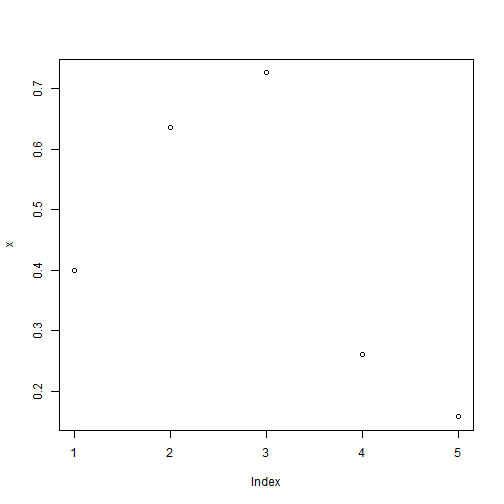``````plot(x, main = "5 random points", xlab = "point number", ylab = "point value")
``````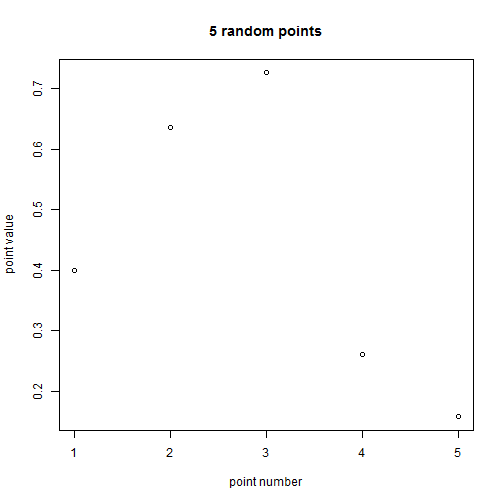``````plot(x, type = "l")  # the same but as line plot
``````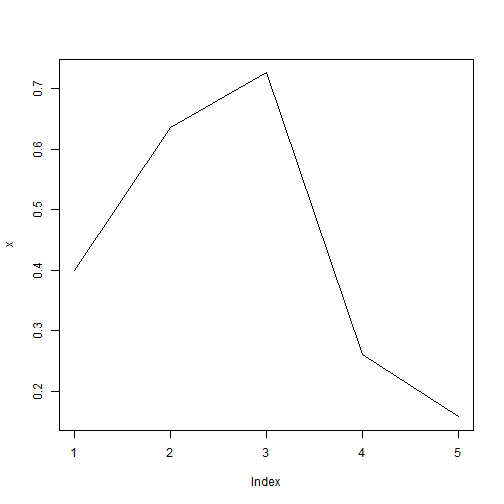``````plot(x, type = "b")  # the same but with both, points and lines
``````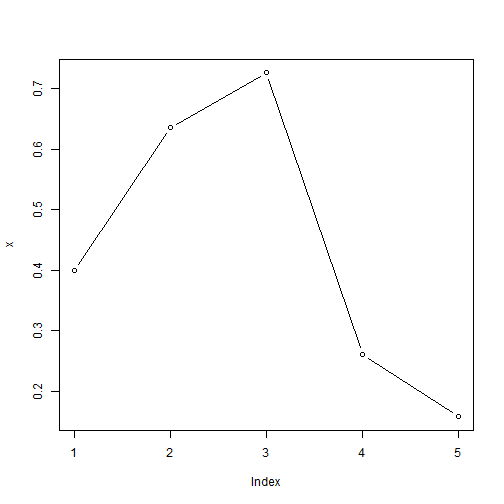``````plot(x, type = "b", col = "blue")  # add some colour
``````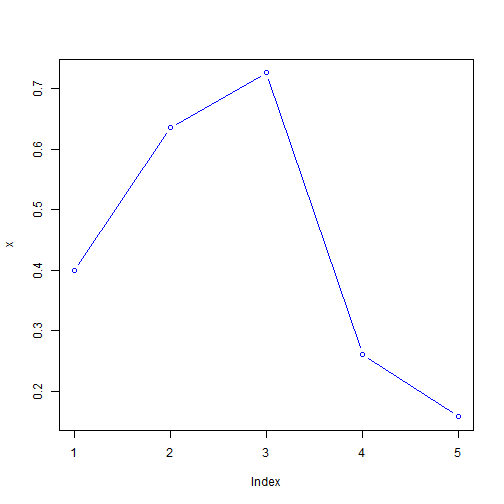``````plot(x, type = "b", col = 1:length(x))  # each point in different colour
``````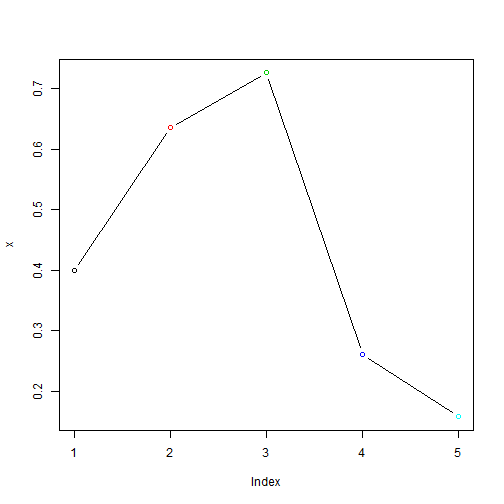``````plot(x, pch = 1:length(x))  # each point as a different symbol
``````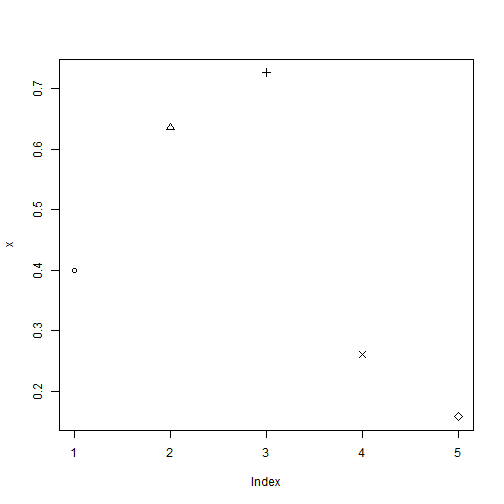Just have a look at the documentation of `plot()` (`?plot`) to see what parameters there are to be tweaked. And this is just a simple one-dimensional plot! There are of course other primitve plots:

``````x <- rnorm(500, 100, 10)  # create 5 normally-distributed random numbers
y <- x^2  # calculate y as the square of x
plot(x, y)  # a scatter plot
``````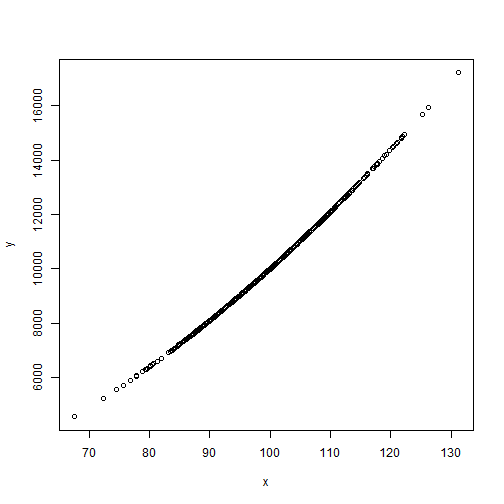``````plot(x, y, log = "xy")  # the same plot but with logarithmic axes
``````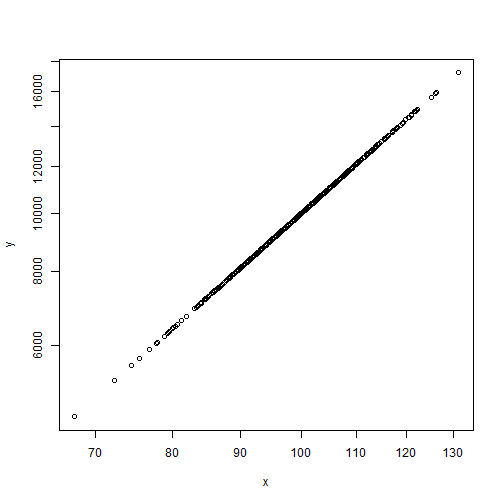``````hist(x)  # a histogram of x
``````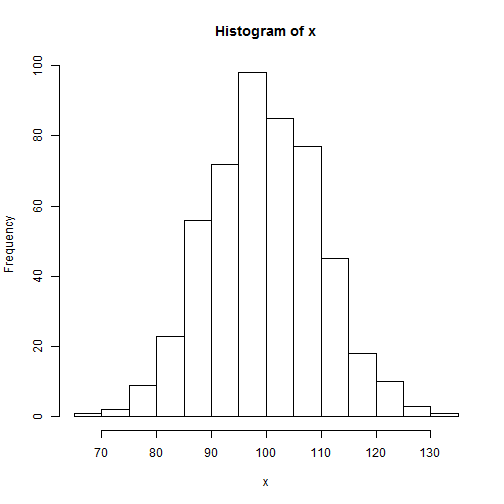``````boxplot(x)  # a boxplot of x
``````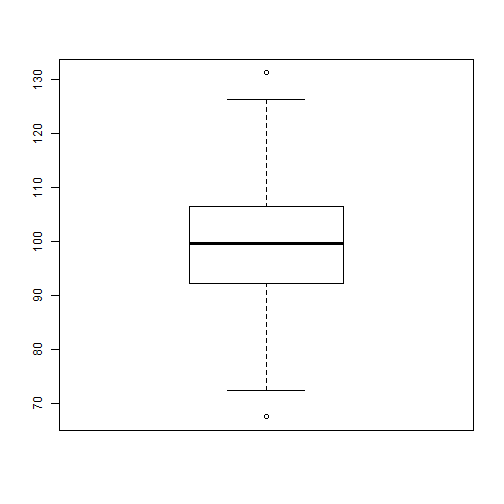And there are many more plots, impossible to present in this short tutorial. To further configure a plot the is the function `par()` which allows formatting the plot more precisely. The function is called prior to the plot function. See `?par` for a long documentation list of possible plot parameters to control for example presence of axes, background and plot colours, line types and widths, number of plots per graphic and plot margins.